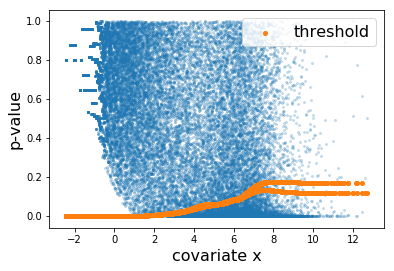Skip to content
{{ message }}

# martinjzhang / adafdr

Switch branches/tags
Nothing to show

## Files

Failed to load latest commit information.
Type
Name
Commit time

## AdaFDRA fast and covariate-adaptive method for multiple hypothesis testing.

## Requirement

• AdaFDR runs on python 3.6 or later

## Installation

``````pip install adafdr
``````

## Version History

• `0.1.8`: latest version.
• The torch dependency was changed to `torch==1.4.0`.
• `scheduler.step()` goes after `optimizer.step()`
• add `loss.backward(retain_graph=True)`
• `0.1.7`: the version released with the paper.

## Usage

### Import package

`adafdr.method` contains all methods while `adafdr.data_loader` contains the data. They can be imported as

```import adafdr.method as md
import adafdr.data_loader as dl```

Other ways of importing are usually compatible. For example, one can import the package with `import adafdr` and call method `xxx` in the method module via `adafdr.method.xxx()`

### Input format

For a set of N hypotheses, the input data includes the p-values `p` and the d-dimensional covariate `x`, with the following format:

• `p`: (N,) numpy.ndarray.
• `x`: (N,d) numpy.ndarray.

When d=1, `x` is allowed to be either (N,) numpy.ndarray or (N,1) numpy.ndarray.

### Covariate visualization

The covariate visualization method `adafdr_explore` can be used as

`adafdr.method.adafdr_explore(p, x, output_folder=None, covariate_type=None)`
• If the `output_folder` is a filepath (str) instead of `None`, the covariate visualization figures will be saved in `output_folder`. Otherwise, they will show up in the console.

• `covariate_type`: a length-d python list with values 0/1. It specifies the type of each covariate: 0 means numerical/ordinal while 1 means categorical. For example, `covariate_type=[0,1]` means there are 2 covariates, the first is numerical/ordinal and the second is categorical. If not specified, a covariate with more than 75 distinct values is regarded as numerical/ordinal and otherwise categorical.

• See also doc for more details.

### Multiple testing

The multiple hypothesis testing method `adafdr_test` can be used as

1. fast version (default, recommended for practical usage):
`res = adafdr.method.adafdr_test(p, x, alpha=0.1, covariate_type=None)`
1. regular version:
`res = adafdr.method.adafdr_test(p, x, alpha=0.1, fast_mode=False, covariate_type=None)`
1. regular version with multi-core:
`res = adafdr.method.adafdr_test(p, x, alpha=0.1, fast_mode=False, single_core=False, covariate_type=None)`
• `res` is a dictionary containing the results, including:
• `res['decision']`: a (N,) boolean vector, decision for each hypothesis with value 1 meaning rejection.
• `res['threshold']`: a (N,) float vector, threshold for each hypothesis.
• If the `output_folder` is a filepath (str) instead of `None`, the logfiles and some intermediate results will be saved in `output_folder`. Otherwise, they will show up in the console.

• `covariate_type`: a length-d python list with values 0/1. It specifies the type of each covariate: 0 means numerical/ordinal while 1 means categorical. For example, `covariate_type=[0,1]` means there are 2 covariates, the first is numerical/ordinal and the second is categorical. If not specified, a covariate with more than 75 distinct values is regarded as numerical/ordinal and otherwise categorical.

• See also doc for more details.

## Example on airway RNA-seq data

The following is an example on the airway RNA-seq data used in the paper.

### Import package and load data

Here we load the airway data used in the paper. See vignettes for other data accompanied with the package.

```import adafdr.method as md
import adafdr.data_loader as dl
p,x = dl.data_airway()```

### Covariate visualization using `adafdr_explore`

`md.adafdr_explore(p, x, output_folder=None)`

Here, the left is a scatter plot of each hypothesis with p-values (y-axis) plotted against the covariate (x-axis). The right panel shows the estimated null hypothesis distribution (blue) and the estimated alternative hypothesis distribution (orange) with respect to the covariate. Here we can conclude that a hypothesis is more likely to be significant if the covariate (gene expression) value is higher.

### Multiple hypothesis testing using `adafdr_test`

`res = md.adafdr_test(p, x, fast_mode=True, output_folder=None)`

Here, the learned threshold `res['threshold']` looks as follows.Each orange dot corresponds to the threhsold to one hypothesis. The discrepancy at the right is due to the difference between the thresholds learned by the two folds.

## Quick Test

Here is a quick test. First check if the package can be successfully imported:

```import adafdr.method as md
import adafdr.data_loader as dl```

Next, run a small example which should take a few seconds:

```import numpy as np
p,x,h,_,_ = dl.load_1d_bump_slope()
res = md.adafdr_test(p, x, alpha=0.1)
t = res['threshold']
D = np.sum(p<=t)
FD = np.sum((p<=t)&(~h))
print('# AdaFDR successfully finished!')
print('# D=%d, FD=%d, FDP=%0.3f'%(D, FD, FD/D))```

It runs AdaFDR-fast on a 1d simulated data. If the package is successfully imported, the result should look like:

``````# AdaFDR successfully finished!
# D=837, FD=79, FDP=0.094
``````

## R API of python package

R API of this package can be found here.

## Citation information

Zhang, Martin J., Fei Xia, and James Zou. "Fast and covariate-adaptive method amplifies detection power in large-scale multiple hypothesis testing." Nature communications 10.1 (2019): 1-11.

Xia, Fei, et al. "Neuralfdr: Learning discovery thresholds from hypothesis features." Advances in Neural Information Processing Systems. 2017.

## About

No description, website, or topics provided.

## Releases 3

0.1.7 release Latest
May 10, 2019

## Packages 0

No packages published

•
•